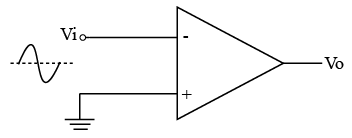# ISRO Scientist or Engineer Electronics 2012

Instructions

For the following questions answer them individually

Question 1

# $$1 cm^{3}$$ of pure Germanium at $$20^\circ$$C contains about $$4.2 \times 10^{22}$$ atoms, $$2.5 \times 10^{13}$$ free electrons and $$2.5 \times 10^{13}$$ holes. 0.001% of Arsenic doping donatesan extra $$10^{17}$$ free electrons in the same volume. The approximate humberof holes in one $$cm^{3}$$ in the doped semiconductor under equilibrium condition is :Question 2

# Measurementof Hall coefficient enables the determination of :Question 3

# Siliconis not suitable for fabrication oflight emitting diodes becauseit is :Question 4

# The band gap of elements arranged in ascendingorderis :Question 5

# The following statements are made for NMOS & PMOS1: The carrier mobility in NMOSis higher2: PMOSrequire less area than NMOS3: NMOS circuits are smaller than PMOS4: PMOS are fasterin switching.Of these, the true statements are -Question 6

# The following statements are made for FETs1. In n-channel depletion mode MOSin the active region, the control voltage Veg is negative.2. NMOSin depletion modeis cut off for $$V_{GS}$$=03. NMOSin enhance modeis cut off for $$V_{GS}$$=04. There is no path betweensource and drain in the enhancement modeOf these, the true statementsare :Question 7

# Special handling precautions should be taken when working with MOS devices. The statement whichis nottrueis :Question 8

# If the input to the circuit of Figure is a sine wave, then the output will be :Question 9

# A high gain OpAmp has $$R_{f} = 10^{5} Ω \& R_{f} = 10^{3} Ω$$. The correct statement is:Question 10

# For various typesofoscillators, the correct statementis:OR